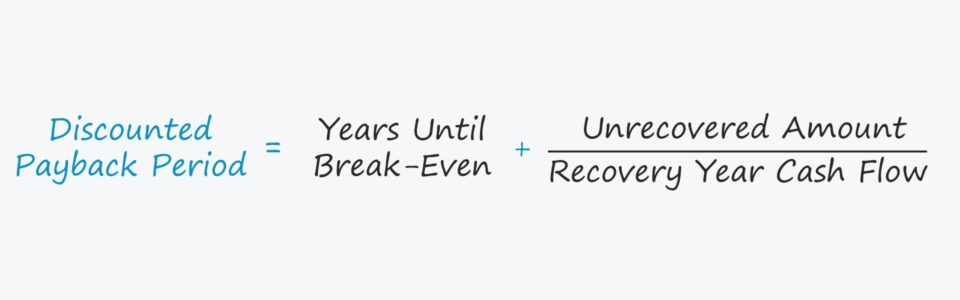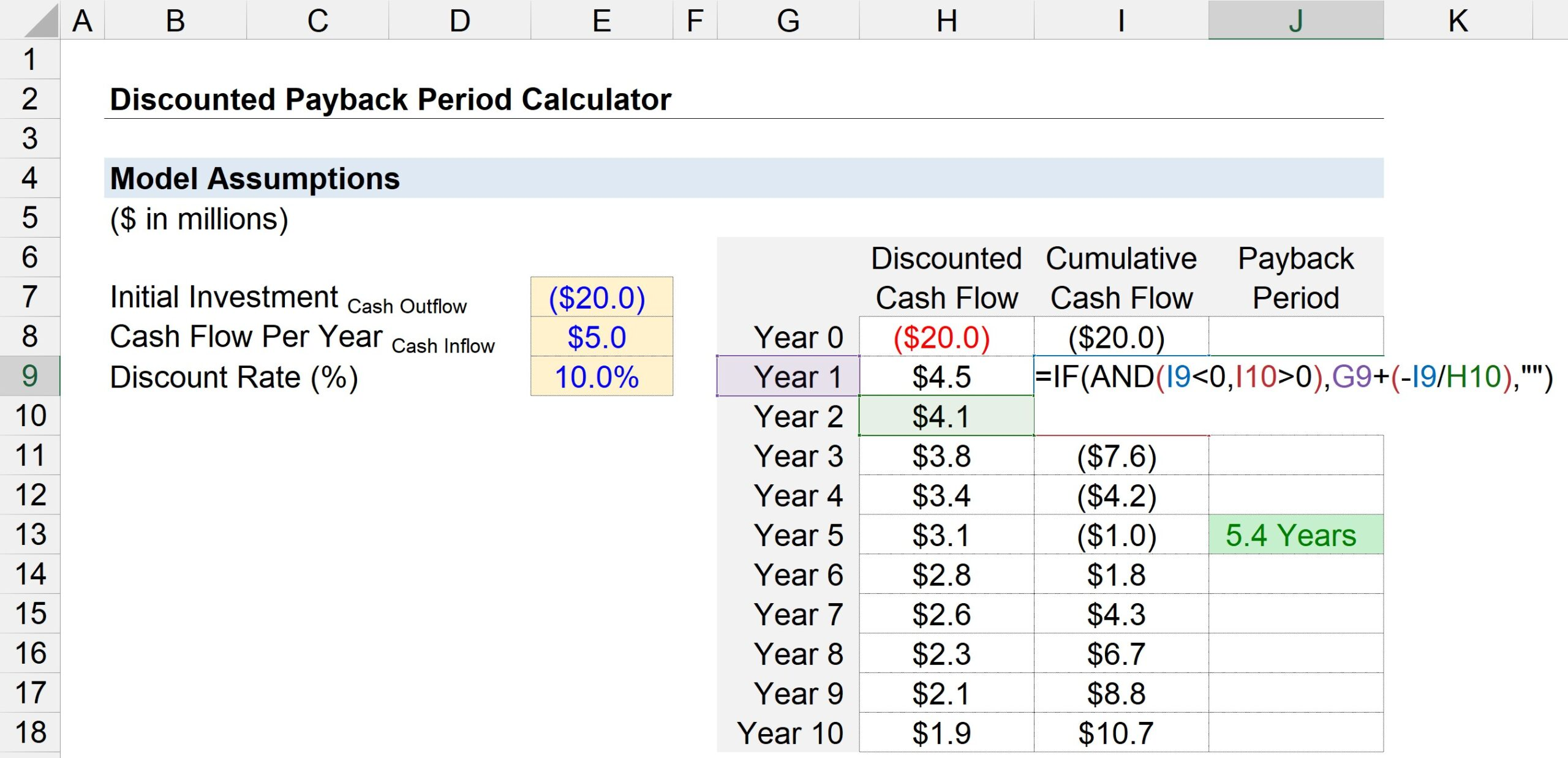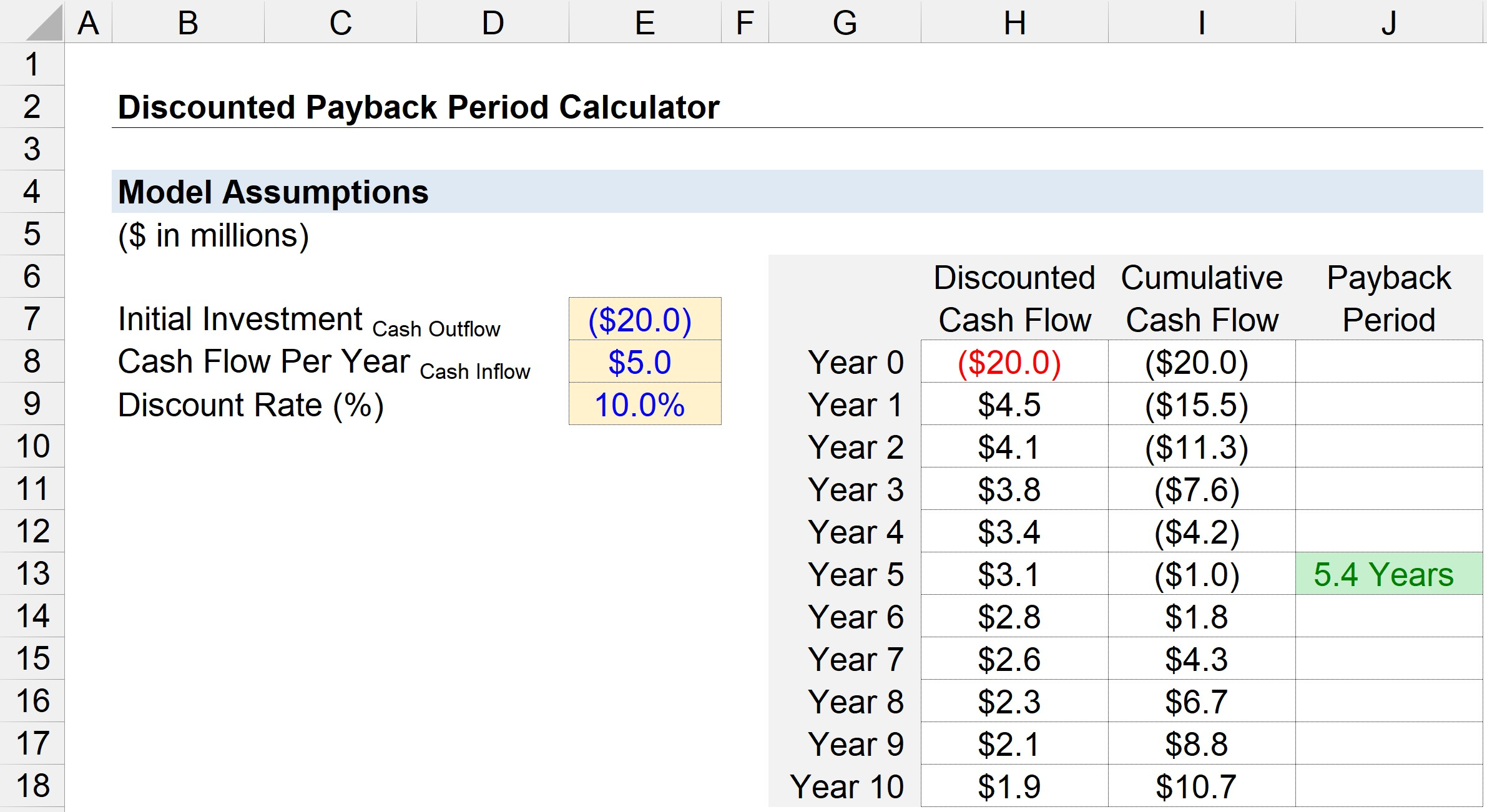Welcome to Wall Street Prep! Use code at checkout for 15% off.# Discounted Payback Period

Understand the Discounted Payback Period Concept## How to Calculate Discounted Payback Period (Step-by-Step)

The shorter the payback period, the more likely the project will be accepted – all else being equal.

In capital budgeting, the payback period is defined as the amount of time necessary for a company to recoup the cost of an initial investment using the cash flows generated by an investment.

Once the payback period is met, the company has reached its break-even point – i.e. the amount of revenue generated by a project is equal to its costs – so beyond the “break-even” threshold, the project is no longer a “loss” to the company.

• Shorter Payback Period → The earlier the cash flows from a project can offset the initial expenditure, the more likely the company will approve the project.
• Longer Payback Period → The more time needed for the project’s cash flows to surpass the initial expenditure, the less likely the project will be approved.

However, one common criticism of the simple payback period metric is that the time value of money is neglected.

Because of the opportunity cost of receiving cash earlier and the ability to earn a return on those funds, a dollar today is worth more than a dollar received tomorrow.

Therefore, it would be more practical to consider the time value of money when deciding which projects to approve (or reject) – which is where the discounted payback period variation comes in.

Calculating the payback period is a two-step process:

• Step 1: Calculate the number of years before the break-even point, i.e. the number of years that the project remains unprofitable to the company.
• Step 2: Divide the unrecovered amount by the cash flow amount in the recovery year, i.e. the cash produced in the period that the company begins to turn a profit on the project for the first time.

## Discounted Payback Period Formula

The formula for computing the discounted payback period is as follows.

Discounted Payback Period = Years Until Break-Even + (Unrecovered Amount / Cash Flow in Recovery Year)

## Simple Payback Period vs. Discounted Method

The formula for the simple payback period and discounted variation are virtually identical.

In fact, the only difference is that the cash flows are discounted in the latter, as is implied by the name.

The implied payback period should thus be longer under the discounted method.

Why? The initial outflow of cash flows is worth more right now, given the opportunity cost of capital, and the cash flows generated in the future are worth less the further out they extend.

The discounted payback period, in theory, is the more accurate measure, since fundamentally, a dollar today is worth more than a dollar received in the future.

In particular, the added step of discounting a project’s cash flows is critical for projects with prolonged payback periods (i.e., 10+ years).

## Discounted Payback Period Calculator – Excel Model Template

We’ll now move to a modeling exercise, which you can access by filling out the form below.Submitting...

## Discounted Payback Period Example Calculation

Suppose a company is considering whether to approve or reject a proposed project.

If undertaken, the initial investment in the project will cost the company approximately \$20 million.

After the initial purchase period (Year 0), the project generates \$5 million in cash flows each year.

Based on the project’s risk profile and the returns on comparable investments, the discount rate – i.e., the required rate of return – is assumed to be 10%.

All of the necessary inputs for our payback period calculation are shown below.

• Initial Investment = –\$20 million
• Cash Flow Per Year = \$5 million
• Discount Rate (%) = 10%

In the next step, we’ll create a table with the period numbers (”Year”) listed on the y-axis, whereas the x-axis consists of three columns.

1. Discounted Cash Flow: In Year 0, we can link to the \$20 million cash outflow, and for all the other years, we can link to the cash flow amount of \$5 million – but remember, we must discount each cash flow by dividing it by one plus the discount rate raised to the period number. Hence, the \$5 million in cash flow amounts to a present value (PV) of \$4.5 million in Year 1 but declines to a PV of \$1.9 million by Year 5.
2. Cumulative Cash Flow: In the next column, we’ll calculate the cumulative cash flow to date by adding the discounted cash flow for the given period to the prior year’s cumulative cash flow balance.
3. Payback Period: The third column uses the “IF(AND)” Excel function to determine the payback period.

More specifically, the logical tests performed are the two shown below:

1. Current Year Cumulative Cash Balance < 0
2. Next Year Cumulative Cash Balance > 0

If both logical tests are true, the break-even occurred somewhere between those two years. However, we are not done here.

Since there is most likely a fractional period that we cannot neglect, the next step is to divide the cumulative cash flow balance as of the current year with a negative placed sign in front of the next year’s cash flow.The two calculated values – the Year number and the fractional amount – can be added together to arrive at the estimated payback period.

The screenshot below shows that the time required to recover the initial \$20 million cash outlay is estimated to be ~5.4 years under the discounted payback period method.Step-by-Step Online Course

### Everything You Need To Master Financial Modeling

Enroll in The Premium Package: Learn Financial Statement Modeling, DCF, M&A, LBO and Comps. The same training program used at top investment banks.